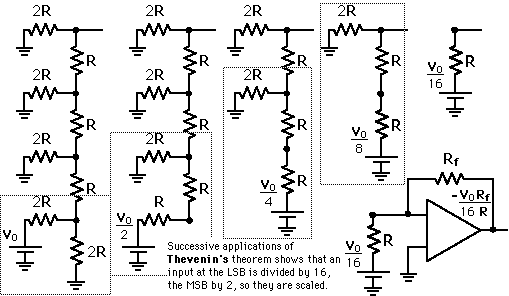# Digital-to-Analog Conversion

When data is in binary form, the 0's and 1's may be of several forms such as the TTL form where the logic zero may be a value up to 0.8 volts and the 1 may be a voltage from 2 to 5 volts. The data can be converted to clean digital form using gates which are designed to be on or off depending on the value of the incoming signal. Data in clean binary digital form can be converted to an analog form by using a summing amplifier. For example, a simple 4-bit D/A converter can be made with a four-input summing amplifier. More practical is the R-2R Network DAC.

 Weighted Summing Amplifier R-2R Network Approach
Index

Electronics concepts

Digital Circuits

 HyperPhysics*****Electricity and magnetism R Nave
Go Back

# Four-Bit D/A Converter

One way to achieve D/A conversion is to use a summing amplifier.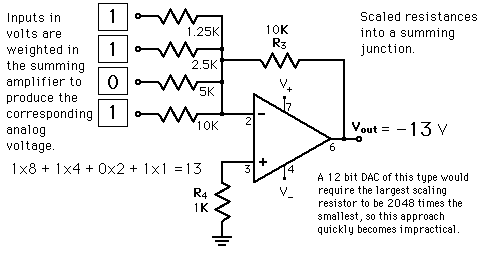This approach is not satisfactory for a large number of bits because it requires too much precision in the summing resistors. This problem is overcome in the R-2R network DAC.
Index

Electronics concepts

Digital Circuits

Reference
Horowitz & Hill
Digital Systems, Sec 9-16.

 HyperPhysics*****Electricity and magnetism R Nave
Go Back

The summing amplifier with the R-2R ladder of resistances shown produces the output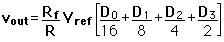where the D's take the value 0 or 1. The digital inputs could be TTL voltages which close the switches on a logical 1 and leave it grounded for a logical 0. This is illustrated for 4 bits, but can be extended to any number with just the resistance values R and 2R.

 More Detail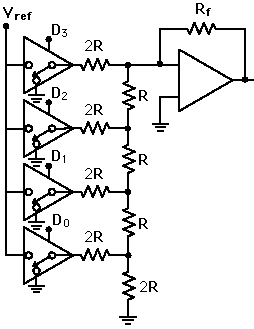Index

Electronics concepts

Digital Circuits

Reference
Horowitz & Hill
Sec 9-16.
Tocci
Digital Systems, Sec 10-3.
Simpson
Sec 15.2.3.

 HyperPhysics*****Electricity and magnetism R Nave
Go Back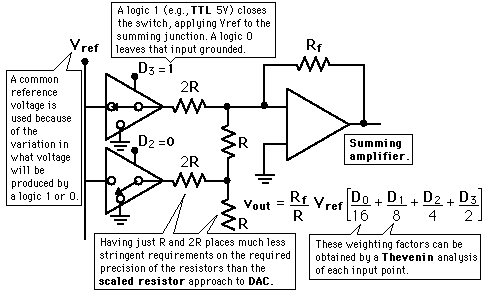TTL Scaled Resistor Thevenin analysis Digital-to-Analog Conversion
Index

Electronics concepts

Diode varieties

Reference
Horowitz & Hill
Sec 9-16.
Tocci
Digital Systems, Sec 10-3.
Simpson
Sec 15.2.3.

 HyperPhysics*****Electricity and magnetism R Nave
Go Back